# Tangrams

The Tangram is a deceptively simple set of seven geometric shapes made up of five triangles (two small triangles, one medium triangle, and two large triangles), a square, and a parallelogram. When the pieces are arranged together they suggest an amazing variety of forms, embodying many numerical and geometric concepts. Tangram pieces are widely used to solve puzzles that require the making of a specified shape using all seven pieces. Tangram sets come in in four colors—red, green, blue, and yellow. The three different-size Tangram triangles are all similar, right isosceles triangles. Thus, the triangles all have angles of 45°, 45°, and 90°, and the corresponding sides of these triangles are in proportion.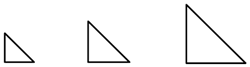Another interesting aspect of the Tangram set is that all of the Tangram pieces can be completely covered with small Tangram triangles.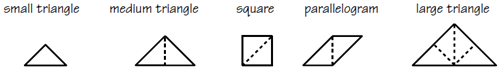Hence, it is easy to see that all the angles of the Tangram pieces are multiples of 45—that is, 45°, 90°, or 135°, and that the small Tangram triangle is the unit of measure that can be used to compare the areas of the Tangram pieces. Since the medium triangle, the square, and the parallelogram are each made up of two small Tangram triangles, they each have an area twice that of the small triangle. The large triangle is made up of four small Tangram triangles and thus has an area four times that of the small triangle and twice that of the other Tangram pieces.

Another special aspect of the pieces is that all seven fit together to form a square.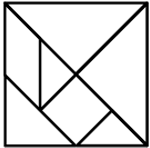Some students can find the making of Tangram shapes to be very frustrating, especially if they are used to being able to do math by following rules and algorithms. For such students, you can reduce the level of frustration by providing some hints. For example, you can put down a first piece, or draw lines on an outline to show how pieces can be placed. However, it is important to find just the right level of challenge so that students can experience the pleasure of each Tangram investigation. Sometimes, placing some Tangram pieces incorrectly and then modeling an exploratory approach such as the following may make students feel more comfortable: "I wonder if I could put this Tangram piece this way. I guess not, because then nothing else can fit here. So I'd better try another way ..."

Tangrams are a good tool for developing spatial reasoning and for exploring fractions and a variety of geometric concepts, including size, shape, congruence, similarity, area, perimeter, and the properties of polygons. Tangrams are especially suitable for students’ independent work, since each student can be given a set for which he or she is responsible. However, since students vary greatly in their spatial abilities and language, time should also be allowed for group work, and most students need ample time to experiment freely with Tangrams before they begin more serious investigations.

Young students will at first think of their Tangram shapes literally. With experience, they will see commonalities and begin to develop abstract language for aspects of patterns within their shapes. For example, students may at first make a square simply from two small triangles. Yet eventually they may develop an abstract mental image of a square divided by a diagonal into two triangles, which will enable them to build squares of other sizes from two triangles.

Tangrams can also provide a visual image essential for developing an understanding of fraction algorithms. Many students learn to do examples such as 1⁄2 = ?⁄8 or 1⁄4 + 1⁄8 + 1⁄16 = ? at a purely symbolic level so that if they forget the procedure, they are at a total loss. Students who have had many presymbolic experiences solving problems such as "Find how many small triangles fill the large triangles," or "How much of the full square is covered by a small, a medium, and a large triangle?" will have a solid intuitive foundation on which to build these basic skills and to fall back on if memory fails them.

Young students have an initial tendency to work with others, and to copy one another's work. Yet, even duplicating someone else's Tangram shape can expand a student’s experience, develop the ability to recognize similarities and differences, and provide a context for developing language related to geometric ideas. Throughout their investigations, students should be encouraged to talk about their constructions in order to clarify and extend their thinking. For example, students will develop an intuitive feel for angles as they fit corners of Tangram pieces together, and they can be encouraged to think about why some pieces will fit in a given space and others won't. Students can begin to develop a perception of symmetry as they take turns mirroring Tangram pieces across a line placed between them on a mat and can also begin to experience pride in their joint production.

Students of any age who haven't seen Tangrams before are likely to first explore shapes by building objects that look like objects—perhaps a butterfly, a rocket, a face, or a letter of the alphabet. Students with a richer geometric background are likely to impose interesting restrictions on their constructions, choosing to make, for example, a filled-in polygon, such as a square or hexagon, or a symmetric pattern.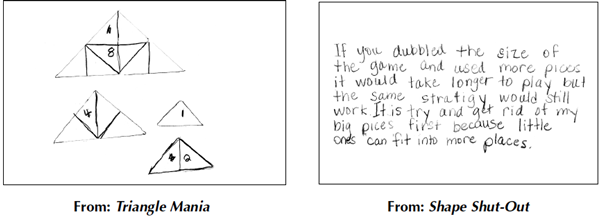The use of Tangrams provides a perfect opportunity for authentic assessment. Watching students work with Tangram pieces gives you a sense of how they approach a mathematical problem. Their thinking can be "seen," in that thinking is expressed through their positioning of the Tangram pieces, and when a class breaks up into small working groups, you are able to circulate, listen, and raise questions, all the while focusing on how individuals are thinking.

To ensure that students know not only how to do a certain operation but also how it relates to a model, assessment should include not only symbolic pencil-and-paper tasks such as "Find 1⁄2 + 1⁄8," but also performance tasks such as "Show why your answer is correct using Tangram pieces."

Having students describe their creations and share their strategies and thinking with the whole class gives you another opportunity for observational assessment. Furthermore, since spatial thinking plays an important role in students’ intellectual development, include in your overall assessment some attention to spatial tasks.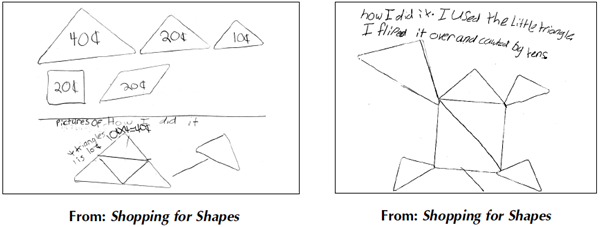## Lessons

The Tangram’s geometry applications are numerous. In addition to creating shapes, younger children can work to fit Tangram pieces into shapes on puzzle cards. Children can gain familiarity with geometric figures while developing spatial reasoning abilities. Spatial tasks may be difficult for some children, but they will improve with experience.

Here are two activities for getting to know Tangrams and to begin using them to learn and understand the math that they represent.

 Activity Curriculum Strands Topics Cover and CountIn this game for two players, children fit Tangram pieces on a dotted grid in an effort to cover as many dots as possible. MeasurementNumberGeometry CountingAdditionCongruenceGame strategies Making a QuiltChildren make paper quilts based on quilt-square patterns they design using Tangram triangles. GeometryPatterns Spatial visualizationLooking for patternsTransformational geometryCongruence

The intermediate grades can use Tangram pieces to create squares, triangles, parallelograms, rectangles, and trapezoids. Students can not only explore and create shapes, they can also learn about relationships among shapes.

Here are two activities for getting to know Tangrams and to begin using them to learn and understand the math that they represent.

 Activity Curriculum Strands Topics Fraction SpinStudents identify a Tangram piece as a fractional part of a whole shape. Then they construct the whole. GeometryNumber Spatial visualizationFractionsCountingComparing Polygon ParadeStudents build polygon shapes using different numbers of Tangram pieces. They record their results on a shape chart. Geometry Spatial visualizationComparingClassifying

Tangrams help students at the middle grades develop spatial-visualization skills and introduce or reinforce geometric concepts such as congruence, similarity, symmetry, etc. Students use them to compute the area of polygons, or Tangrams can be used to introduce the terms “congruent” and “similar.”

Here are two activities for getting to know Tangrams and to begin using them to learn and understand the math that they represent.

 Activity Curriculum Strands Topics Square Cover-UpStudents cover a grid with Tangram pieces and find the percent of the grid that is covered. NumberMeasurement PercentsEstimationArea The More, The BetterStudents search for all the convex Tangram shapes that can be made with different numbers of pieces that represent the same fractional part of the seven-piece Tangram square. GeometryNumber ComparingClassifyingPolygonsFractional equivalenceSpatial visualization

### Skills Taught

• Analyzing Data
• Angles
• Area
• Chance
• Circle Graphs & Central Angles
• Classifying
• Combinations
• Comparing
• Congruence
• Equivalent Fractions
• Estimating
• Experimental Probability
• Following Directions
• Fractional Equivalence
• Game Strategies
• Geometry Patterns
• Graphing Properties of Polygons
• Language of Mathematics
• Looking for Patterns
• Measures of Central Tendency
• Nonstandard Measurement
• Number
• Patterns/Functions
• Perimeter
• Permutations
• Polygons
• Properties of Angles
• Properties of Geometric Figures
• Properties of Polygons
• Properties of Triangles
• Proportion
• Ratio
• Scale Drawings
• Shape Fitting
• Similar Polygons
• Similarity
• Spatial Visualization
• Standard Measurements
• Symmetry
• Theoretical Probability
• Transformational Geometry